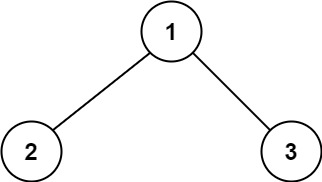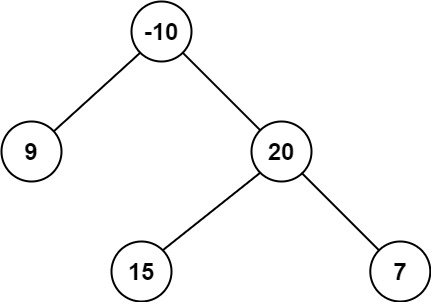# LeetCode - #124 二叉樹中的最大路徑和（Top 100）

## 前言

LeetCode 演算法到目前我們已經更新到 123 期，我們會保持更新時間和進度（週一、週三、週五早上 9:00 釋出），每期的內容不多，我們希望大家可以在上班路上閱讀，長久積累會有很大提升。

## 2. 示例```輸入：root = [1,2,3] 輸出：6 解釋：最優路徑是 2 -> 1 -> 3 ，路徑和為 2 + 1 + 3 = 6``````輸入：root = [-10,9,20,null,null,15,7] 輸出：42 解釋：最優路徑是 15 -> 20 -> 7 ，路徑和為 15 + 20 + 7 = 42```

• 樹中節點數目範圍是 `[1, 3 * 10^4]`
• `-1000 <= Node.val <= 1000`

## 3. 答案

```swift / * Definition for a binary tree node. * struct TreeNode { * int val; * TreeNode left; * TreeNode right; * TreeNode() : val(0), left(nullptr), right(nullptr) {} * TreeNode(int x) : val(x), left(nullptr), right(nullptr) {} * TreeNode(int x, TreeNode left, TreeNode right) : val(x), left(left), right(right) {} * }; */

class BinaryTreeMaximumPathSum {

``````var maxValue = Int.min

func maxPathSum(_ root: TreeNode?) -> Int {
maxWeight(root)
return maxValue
}

private func maxWeight(_ node: TreeNode?) -> Int {
guard let node = node else {
return 0
}

let leftMaxWeight = max(maxWeight(node.left), 0)
let rightMaxWeight = max(maxWeight(node.right), 0)

maxValue = max(maxValue, leftMaxWeight + node.val + rightMaxWeight)

return node.val + max(leftMaxWeight, rightMaxWeight)
}
``````

} ```

• 主要思想：迭代所有樹節點，計算每個節點的最大權值，並在迭代過程中更新最大路徑和。
• 時間複雜度： O(n)
• 空間複雜度： O(1)